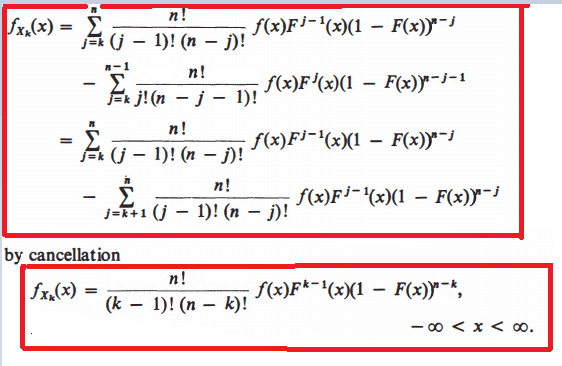# Derivation of P.D.F. from distribution function

• A
• WMDhamnekar

#### WMDhamnekar

MHB
TL;DR Summary
If ## F_{X_k}(x) = p(X \leq x) = \displaystyle\sum_{j=k}^n \binom{n}{j} F^j(x)(1-F(x))^{n-j}, -\infty
< x < \infty ## then how to prove ##f_{X_k} (x) =\frac{n!}{(k-1)!(n-k)!}f(x) F^{k-1}(x)(1-F(x))^{n-k}##
Author computed ##f_{X_k}(x)## as follows but I don't understand it. Would any member explain me the following computations?